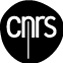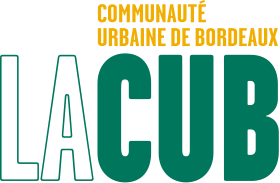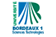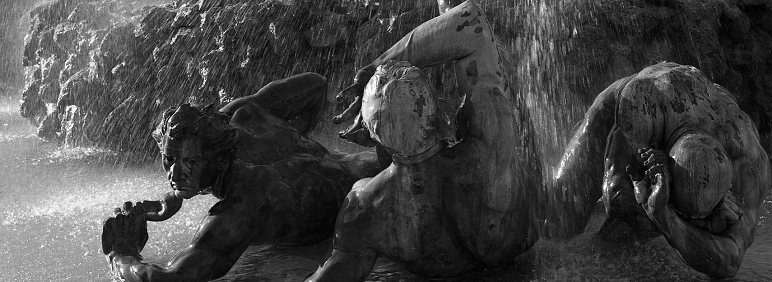Bordeaux Graph Workshop
Bordeaux, November 21-24, 2012.
LaBRI, Université Bordeaux 1, 351 cours de la libération, Talence, France.
 > BGW 2012 is the second edition of Bordeaux Graph Workshop. Organized by the "Graphs and Applications" group.### Program

This is a preliminary program: here. (last modification: November, 17).
Here is the booklet (last modification: November, 14).

### Presentation guidelines

Oral contributed presentations are given a 25 minutes slot:

• please make sure your presentation lasts at most 20 minutes. The 5 extra minutes are reserved for discussing and switching speaker.
• please bring the slideshow for your presentation in a pdf file on a flashdisk at the beginning of the session, in order to upload them on the room laptop. We cannot guarantee that other slideshow file types would be read properly.
• there are small parts of whiteboard next to the screen for illustrating your talk, yet do not rely too much on it for your presentation, it is rather inconvenient.

### Abstracts of plenary talks

Finding a Subdivision of a Digraph. - F. Havet

We consider the following problem:
Given a directed graph D, does it contain a subdivision of a prescribed digraph F? We give a number of examples of polynomial instances, several NP-completeness proofs as well as a number of conjectures and open problems.

This is joint work with J. Bang-Jensen and A.-K. Maia.

Extending partial representations of graphs - J. Kratochvil

We study the computational complexity of the following general question: Given a graph class defined by geometric representations of a certain type, and given a graph and a representation of some of its vertices, can this be extended to a representation of the entire graph? This falls into a general paradigm of extending a partial solution of a problem to a full one which was studied in various connections (coloring, noncrossing drawing etc.). In many cases the extension problem is provably harder which corresponds to a common knowledge of architects - building from scratch is easier than restoring an old house. The results we will report upon include extension of geometrically defined classes of intersection graphs - interval graphs, permutation graphs, function graphs, and chordal graphs. Perhaps slightly suprisingly we will see that in most cases the extension problem remains polynomial.

Playing for Time: 1101110 years of Combinatorial Game Theory - R. Nowakowski

Combinatorial Game Theory (CGT) is a new mathematical subject despite being a very old human endeavour. I'll give a brief, admittedly biased, overview of the ideas (such as Hot games, incentives, atomic weight, flowers, miserable monoids), problems, and people, from Bouton's 1902 paper on NIM to the first comprehensive CGT text (soon-to-be-published by Aaron Siegel). The talk will assume no specialist knowledge of CGT.

The Cycle Spectrum of Graphs - D. Rautenbach

Cycles in graphs and especially their lengths are among the most well-studied topics in graph theory. The set of all cycle lengths of a graph is known as its cycle spectrum. We present recent results concerning

• the cycle spectrum of Hamiltonian graphs,
• the cycle spectrum of squares of graphs,
• a short proof of a versatile version of Fleischner's well-known theorem, and
• Ramsey-type results for the cycle spectrum.
The talk is based on joint work with S. Brandt, F. Joos, D. Meierling, K. Milans, J. Müttel, F. Regen, T. Sasse, D. West.

Graphs, Tournaments, Colouring and Containment - P. Seymour

Some tournaments H are ``heroes''; they have the property that all tournaments not containing H as a subtournament have bounded chromatic number (colouring a tournament means partitioning its vertex-set into transitive subsets). In joint work with eight authors, we found all heroes explicitly. That was great fun, and it would be nice to find an analogue for graphs instead of tournaments.
The problem is too trivial for graphs, if we only exclude one graph H; but it becomes fun again if we exclude a finite set of graphs. The Gyarfas-Sumner conjecture says that if we exclude a forest and a clique then chromatic number is bounded. So what other combinations of excluded subgraphs will give bounded chromatic (or cochromatic) number? It turns out (assuming the Gyarfas-Sumner conjecture) that for any finite set S of graphs, the graphs not containing any member of S all have bounded cochromatic number if and only if S contains a complete multipartite graph, the complement of a complete multipartite graph, a forest, and the complement of a forest.
Proving this led us to the following: for every complete multipartite graph H, and every disjoint union of cliques J, there is a number n with the following property. For every graph G, if G contains neither of H,J as an induced subgraph, then V(G) can be partitioned into two sets such that the first contains no n-vertex clique and the second no n-vertex stable set.
In turn, this led us (with Alex Scott) to the following stronger result. Let H be the disjoint union of H_1,H_2, and let J be obtained from the disjoint union of J_1,J_2 by making every vertex of J_1 adjacent to every vertex of J_2. Then there is a number n such that for every graph G containing neither of H,J as an induced subgraph, V(G) can be partitioned into n sets such that for each of them, say X, one of H_1,H_2,J_1,J_2 is not contained in G|X.
How about a tournament analogue of this? It exists, and the same (short) proof works; and this leads to a short proof of the most difficult result of the heroes paper that we started with. There are a number of other related results and open questions. Joint work with Maria Chudnovsky.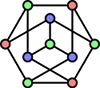ANR DORSO
ANR GRATOS
ANR GRATEL
ANR IDEA
CNRS Chaire
Communauté Urbaine de Bordeaux
Conseil Régional
INRIA
IUF
LaBRI
Maths ŕ Modeler
Office du tourisme de Bordeaux
Région Aquitaine
Université Bordeaux 1